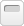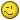• Jueves 28 de Septiembre de 2023, 23:31

###Autor Tema:  Rotación De Imágenes En Delphi.  (Leído 2027 veces)

#### Amilius

• Miembro HIPER activo
••• Mensajes: 665##### Rotación De Imágenes En Delphi.
« en: Domingo 5 de Septiembre de 2004, 17:08 »
0
Adjunto una carta de respuesta conteniendo el código en el buen DELPHI para realizar rotación con antialisado. Si alguien consigue un componente para delphi o mejor un método para una clase heredada del Tbitmap sea bienvenido!!!.

Verán que el nivel de complejidad de la operación a nivel de pixel es elevado, un montón de multiplicaciones y saltos (if).Código: Text
1.
2. From: Harm &#60;harmans@uswest.net&#62;
3. Subject: Re: 2d rotaion trouble!
4. Date: 28 Mar 1999 00:00:00 GMT
5. Message-ID: &#60;36FE5D74.C74E566C@uswest.net&#62;
6. Content-Transfer-Encoding: 7bit
7. References: &#60;e5bL2.456&#036;Xc6.578@news.get2net.dk&#62;
8. Content-Type: text/plain; charset=us-ascii
9. X-Complaints-To: harmans@uswest.net
10. NNTP-Posting-Date: Sun, 28 Mar 1999 10:48:22 CDT
11. Newsgroups: comp.graphics.algorithms
12.
13. Here's Delphi pascal code to rotate an image any angle, with edge wrapping,
14. and anti-aliasing.  Converted and added the edge wrapping, from original VB
15. code by Rod Stephens.  At least it wasn't (shudder) C++.  :)
16.
17. procedure TForm1.RotateAngle(Angle: integer);
18. var
19.   Theta, cosTheta, sinTheta: Single;
20.     cx, cy                 : Single; //Center X, Y
21.     sfrom_y, sfrom_x       : Single; //Real number
22.     ifrom_y, ifrom_x       : Integer; //Integer version
23.     to_y, to_x             : Integer;
24.     weight_x, weight_y     : array[0..1] of Single;
25.     weight                 : Single;
26.     new_red, new_green     : Integer;
27.     new_blue               : Integer;
28.     total_red, total_green : Single;
29.     total_blue             : Single;
30.     ix, iy                 : Integer;
31.     pb, pc : pRGBArray;
32. begin
33.   Screen.Cursor := crHourGlass;
34.   CopyMe(ud,b);  //Copy to the undo bitmap
35.   CopyMe(tBufr,b);  //Copy to the internal buffer bitmap
36.     // Calculate the sine and cosine of theta for later.
37.   Theta:=-(Angle)*Pi/180;
38.   sinTheta:=Sin(Theta);
39.   cosTheta:=Cos(Theta);
40.   cx := b.Width / 2;  //Center of rotation for x
41.   cy := b.Height / 2; //Center of rotation for y
42.     // Perform the rotation.
43.   for to_y := 0 to b.Height-1 do begin
44.     for to_x := 0 to b.Width-1 do begin
45.             // Find the location (from_x, from_y) that
46.             // rotates to position (to_x, to_y).
47.       sfrom_x := cx +
48.           (to_x - cx) * cosTheta -
49.           (to_y - cy) * sinTheta;
50.       ifrom_x := Trunc(sfrom_x);
51.
52.       sfrom_y := cy +
53.           (to_x - cx) * sinTheta +
54.           (to_y - cy) * cosTheta;
55.       ifrom_y := Trunc(sfrom_y);
56.
57.                 // Calculate the weights.
58.       if sfrom_y &#62;= 0  then begin
59.         weight_y := sfrom_y - ifrom_y;
60.         weight_y := 1 - weight_y;
61.       end else begin
62.         weight_y := -(sfrom_y - ifrom_y);
63.         weight_y := 1 - weight_y;
64.       end;
65.       if sfrom_x &#62;= 0 then begin
66.         weight_x := sfrom_x - ifrom_x;
67.         weight_x := 1 - weight_x;
68.       end else begin
69.         weight_x := -(sfrom_x - ifrom_x);
70.         Weight_x := 1 - weight_x;
71.       end;
72.
73.       if ifrom_x &#60; 0 then
74.         ifrom_x := b.Width -1-(-ifrom_x mod b.Width)
75.       else if ifrom_x &#62; b.Width-1  then
76.         ifrom_x := ifrom_x mod b.Width;
77.       if ifrom_y &#60; 0 then
78.         ifrom_y := b.Height -1-(-ifrom_y mod b.Height)
79.       else if ifrom_y &#62; b.Height-1 then
80.         ifrom_y := ifrom_y mod b.Height;
81.
82.                 // Average the color components of the four
83.                 // nearest pixels in from_canvas.
84.         total_red   := 0.0;
85.         total_green := 0.0;
86.         total_blue  := 0.0;
87.         for ix := 0 to 1 do begin
88.           for iy := 0 to 1 do begin
89.             if ifrom_y + iy &#60; b.Height then
90.               pc := tBufr.ScanLine[ifrom_y + iy]
91.             else
92.               pc := tBufr.ScanLine[b.Height - ifrom_y - iy];
93.             if ifrom_x + ix &#60; b.Width then begin
94.               new_red := pc[ifrom_x + ix].rgbtRed;
95.               new_green := pc[ifrom_x + ix].rgbtGreen;
96.               new_blue := pc[ifrom_x + ix].rgbtBlue;
97.               weight := weight_x[ix] * weight_y[iy];
98.               total_red   := total_red   + new_red   * weight;
99.               total_green := total_green + new_green * weight;
100.               total_blue  := total_blue  + new_blue  * weight;
101.             end
102.             else begin
103.               new_red := pc[b.Width - ifrom_x - ix].rgbtRed;
104.               new_green := pc[b.Width - ifrom_x - ix].rgbtGreen;
105.               new_blue := pc[b.Width - ifrom_x - ix].rgbtBlue;
106.               weight := weight_x[ix] * weight_y[iy];
107.               total_red   := total_red   + new_red   * weight;
108.               total_green := total_green + new_green * weight;
109.               total_blue  := total_blue  + new_blue  * weight;
110.             end;
111.           end;
112.         end;
113.         pb := b.ScanLine[to_y];
114.         pb[to_x].rgbtRed := Round(total_red);
115.         pb[to_x].rgbtGreen := Round(total_green);
116.         pb[to_x].rgbtBlue := Round(total_blue);
117.     end;
118.   end;
119.   imSine.Picture.Assign(b);  //all done, assign the bmp to our image
120.   Screen.Cursor := crDefault;
121. end;
122.
123.
124. morphix wrote:
125.
126. &#62; I have some problems figuring how to do a 2d rotation on an image. The
127. &#62; resulting image has blank pixels (holes that isn't filled with color)
128. &#62; could anybody please show me an algorithm that works, (pascal, c, c++ or
129. &#62; pseudo)
130. &#62;
131. &#62; Morphix
132.
133. --
134. Harmans
135.
136. Omaha NE
137.
138.